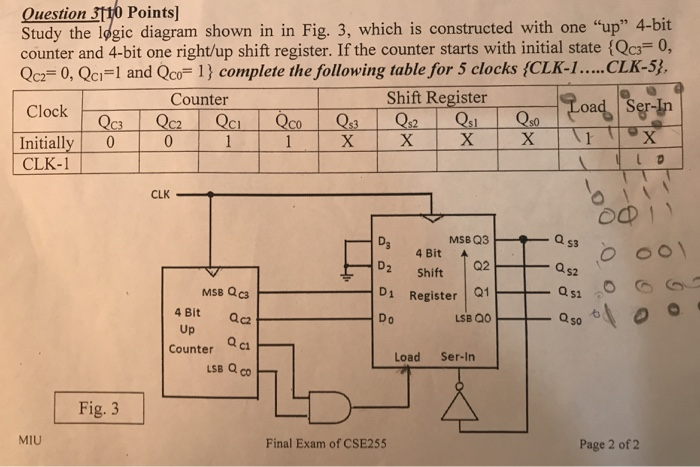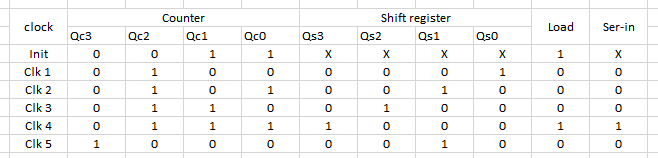# Question & Answer: er. If the counter starts with initial state {Q_C1 = 0, Q_C2 = 0, Q_C1 = 1 and Q_C0 =…..Study the logic diagram shown in Fig. 3, which is constructed with one “up” 4-bit counter and 4-bit one right/up shift register. If the counter starts with initial state {Q_C1 = 0, Q_C2 = 0, Q_C1 = 1 and Q_C0 = 1} complete the following table for 5 clocks (CLK-1 CLK-5}.